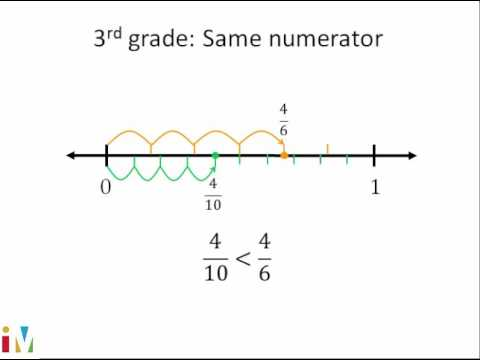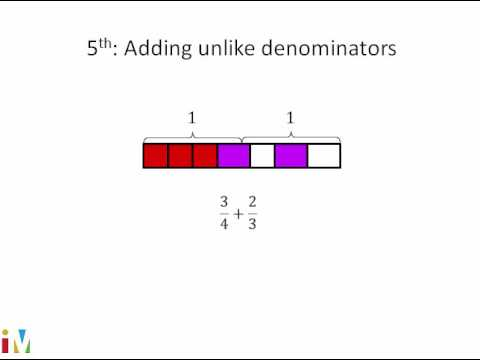Video

# Dividing fractions by whole numbers: studying (Full video)

Description: This video shows how to solve a word problem by dividing a fraction by a whole number (in this case, 1/5 ÷ 4). So the total amount of time he's going to spend studying this weekend is 1/5 of the weekend. And he's going to spend that much time on each subject. Now, we've already seen that dividing by a number is the same thing as multiplying by its reciprocal.

### Other videos you might be interested in### Comparing Fractions

#### Illustrative Mathematics